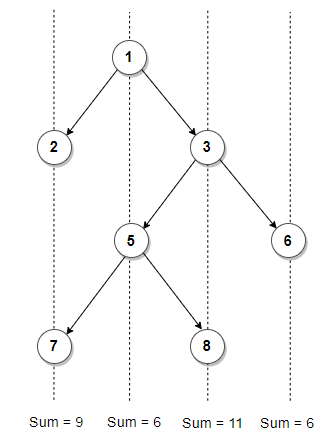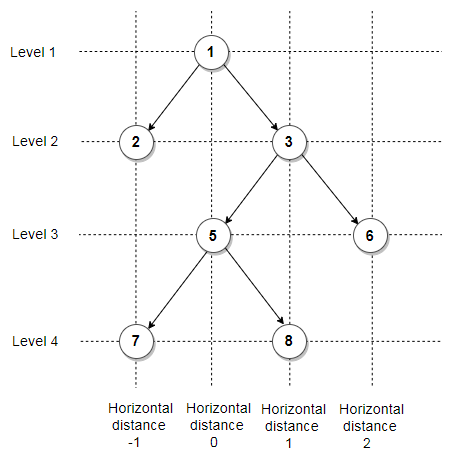# Find Vertical Sum in a given Binary Tree

Given a binary tree, print vertical sum of it. Assume, the left and right child of a node makes 45 degree angle with the parent.

For example, vertical sum is shown in below binary tree### 1. Using Hashing

This problem can be easily solved with the help of Hashing. The idea is to create an empty map where each key represents the relative horizontal distance of a node from the root node and value in the map maintains sum of all nodes present at same horizontal distance. Then we do a pre-order traversal of the tree and we update the sum for current horizontal distance in the map. For each node, we recurse for its left subtree by decreasing horizontal distance by 1 and recurse for right subtree by increasing horizontal distance by 1.

Below figure shows horizontal distance and level of each node in above binary tree. The final values in the map will be

(horizontal distance -> vertical sum)

-1 -> 9
0 -> 6
1 -> 11
2 -> 6Here’s a C++ program that demonstrates it:

Output:

9 6 11 6

The time complexity of above solution is O(nlog(n)) and auxiliary space used by the program is O(n).

### 2. Using Auxiliary Data Structure

We can improve time complexity of above program to linear by using doubly linked list data structure. The idea here is to store vertical sum of the binary tree in a doubly linked list where each node of doubly linked list stores the sum of all nodes corresponding to a vertical line in a binary tree.

We start by constructing a doubly linked list node which stores sum of nodes present at vertical line passing through the root node. Then node->prev and node->next will correspond to the sum of nodes present at vertical line passing through the left and right child of the root node respectively. The trick is to recursively construct the linked list and update nodes with the vertical sums as we traverse the tree.

The algorithm can be implemented as follows in C++:

Output:

9 6 11 6

The time complexity of above solution is O(n) and auxiliary space used by the program is O(n) for linked list nodes.(1 votes, average: 5.00 out of 5)Loading...